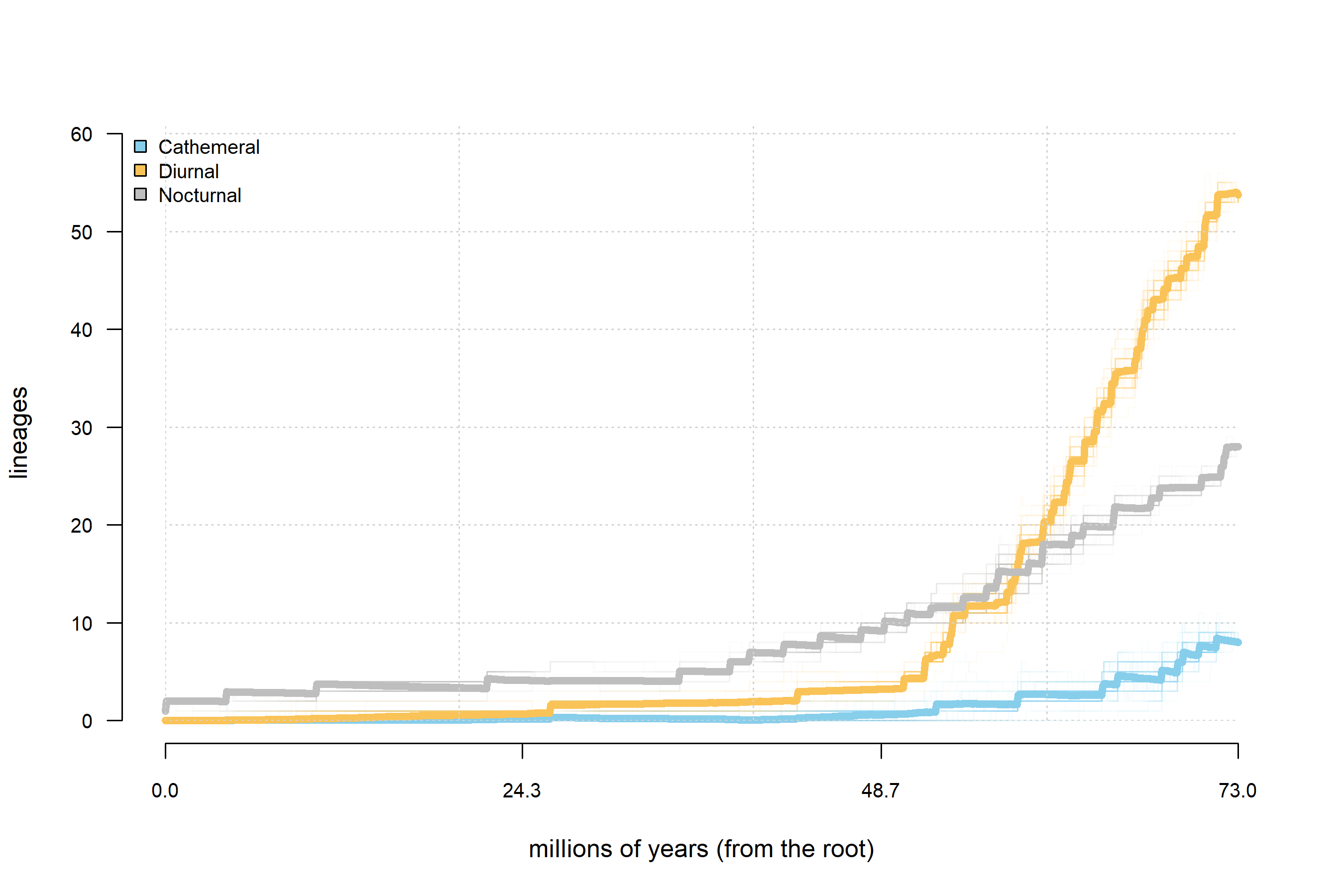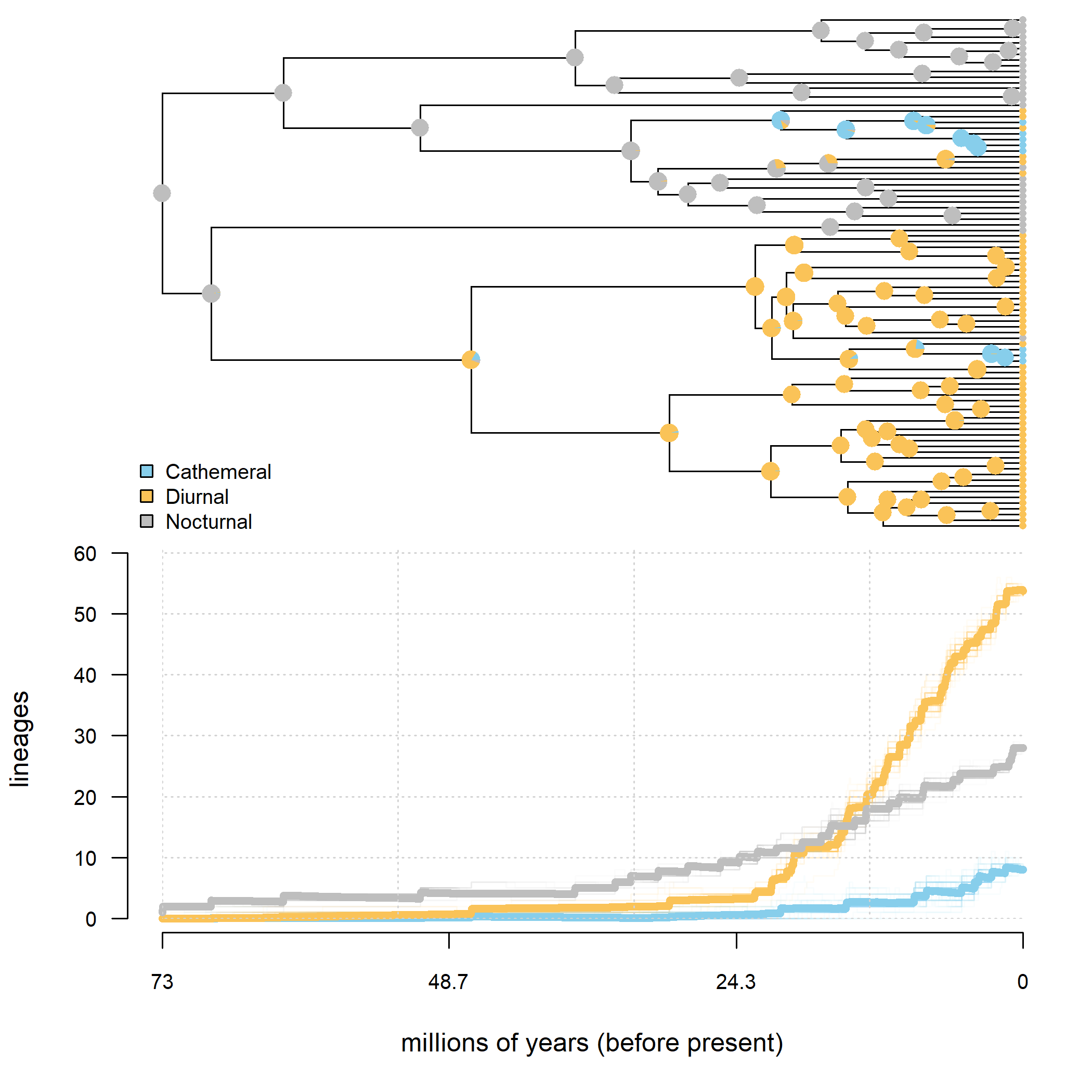## Monday, August 1, 2022

### Lineages-through-time plots for a `"multiSimmap"` object

Earlier today I posted about new S3 methods in phytools to compute and plot lineages-through-time from a single stochastic character mapped tree.

Generally, however, we're not analyzing a single stochastic character map, but a set of such maps sampled in proportion to their probability under a model. I've now extended what I showed earlier to a `"multiSimmap"` object as well. I'll demontrate, but to follow along users will need to update phytools from its GitHub page, which I recommend doing using the CRAN package devtools (and as I showed in my earlier post).

To illustrate this method, I'll use some data for primate diel activity pattern from Kirk and Kay (2004), that also features (in an entirely different context) in my upcoming book (already available for Kindle and other e-readers) with Luke Harmon.

We can start by loading phytools – and, remember, for this to work, your phytools package version should match or be higher than the version number indicated below.

``````library(phytools)
packageVersion("phytools")
``````
``````##  '1.1.7'
``````

Now let's read our tree and data from file as follows.

``````primate.tree<-read.tree(file="http://www.phytools.org/Rbook/3/primateEyes.phy")
primate.tree
``````
``````##
## Phylogenetic tree with 90 tips and 89 internal nodes.
##
## Tip labels:
##   Allenopithecus_nigroviridis, Cercopithecus_mitis, Cercopithecus_cephus, Cercopithecus_petaurista, Erythrocebus_patas, Chlorocebus_aethiops, ...
##
## Rooted; includes branch lengths.
``````
``````primate.data<-read.csv(file="http://www.phytools.org/Rbook/3/primateEyes.csv",
row.names=1,stringsAsFactors=TRUE)
``````
``````##                                  Group Skull_length Optic_foramen_area Orbit_area Activity_pattern
## Allenopithecus_nigroviridis Anthropoid         98.5                7.0      298.7          Diurnal
## Alouatta_palliata           Anthropoid        109.8                5.3      382.3          Diurnal
## Alouatta_seniculus          Anthropoid        108.0                8.0      359.4          Diurnal
## Aotus_trivirgatus           Anthropoid         60.5                3.1      297.4        Nocturnal
## Arctocebus_aureus            Prosimian         49.5                1.2      134.8        Nocturnal
## Arctocebus_calabarensis      Prosimian         53.8                1.6      156.6        Nocturnal
##                             Activity_pattern_code
## Allenopithecus_nigroviridis                     0
## Alouatta_palliata                               0
## Alouatta_seniculus                              0
## Aotus_trivirgatus                               2
## Arctocebus_aureus                               2
## Arctocebus_calabarensis                         2
``````

We can extract the variable that we're interested in, in this case, `Activity_pattern`.

``````Activity_pattern<-setNames(primate.data\$Activity_pattern,
rownames(primate.data))
``````

Now we're ready to do our stochastic mapping. Here, I'll do this under the ARD (all-rates-different) model. (But in a real study, we might use model selection to choose the model that is best-supported by our data.)

``````primate.maps<-make.simmap(primate.tree,Activity_pattern,model="ARD",
pi="fitzjohn",nsim=100)
``````
``````## make.simmap is sampling character histories conditioned on
## the transition matrix
##
## Q =
##              Cathemeral      Diurnal    Nocturnal
## Cathemeral -0.050529521  0.050529521  0.000000000
## Diurnal     0.002306398 -0.004367451  0.002061052
## Nocturnal   0.002586387  0.003565036 -0.006151422
## (estimated using likelihood);
## and (mean) root node prior probabilities
## pi =
##  Cathemeral     Diurnal   Nocturnal
## 0.003675779 0.021703763 0.974620458
``````
``````## Done.
``````
``````primate.maps
``````
``````## 100 phylogenetic trees with mapped discrete characters
``````

OK, now let's compute our LTTs.

``````primate.ltts<-ltt(primate.maps)
primate.ltts
``````
``````## 100 objects of class "ltt.simmap" in a list
``````

We can set the colors that we'd like to use for plotting.

``````cols<-setNames(c("#87CEEB","#FAC358","gray"),
levels(Activity_pattern))
``````

Finally, let's create our plot.

``````plot(primate.ltts,colors=cols,show.total=FALSE,bty="n",cex.axis=0.8,
las=1,xlab="millions of years (from the root)",axes=FALSE)
axis(1,at=round(seq(0,max(nodeHeights(primate.tree)),length.out=4),1),
cex.axis=0.8)
axis(2,las=1,cex.axis=0.8)
clip(0,max(nodeHeights(primate.tree)),0,Ntip(primate.tree))
grid()
``````Cool.

Lastly, here is the same thing, but also combined with a graph of our phylogeny showing the posterior probabilities for each node.

``````obj<-summary(primate.maps)
par(mfrow=c(2,1))
plotTree(primate.tree,ftype="off",lwd=1,mar=c(0,4.1,0,1.1))
legend("bottomleft",levels(Activity_pattern),pch=22,
pt.bg=cols,cex=0.8,pt.cex=1.2,bty="n")
par(fg="transparent")
nodelabels(pie=obj\$ace,piecol=cols,cex=0.5)
tiplabels(pie=to.matrix(Activity_pattern,
levels(Activity_pattern))[primate.tree\$tip.label,],
piecol=cols,cex=0.2)
par(fg="black")
par(mar=c(5.1,4.1,0,1.1))
plot(primate.ltts,colors=cols,show.total=FALSE,bty="n",cex.axis=0.8,
las=1,xlab="millions of years (before present)",axes=FALSE,
legend=FALSE)
axis(1,at=round(seq(0,max(nodeHeights(primate.tree)),length.out=4),1),
label=round(seq(max(nodeHeights(primate.tree)),0,length.out=4),1),
cex.axis=0.8)
axis(2,las=1,cex.axis=0.8)
clip(0,max(nodeHeights(primate.tree)),0,Ntip(primate.tree))
grid()
``````Oooh. That's awesome.